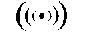# Plucked-String Synthesis Algorithms with Tension Modulation Nonlinearity

### Vesa Välimäki, Tero Tolonen, and Matti Karjalainen

Helsinki University of Technology, Laboratory of Acoustics and Audio Signal Processing, P.O. Box 3000, FIN-02015 HUT, Espoo, Finland

### ABSTRACT

Digital waveguide modeling of a nonlinear vibrating string is investigated when the nonlinearity is essentially caused by tension modulation. We derive synthesis models where the nonlinearity is implemented with a time-varying fractional delay filter. Also, conversion from a dual-delay-line physical model into a single-delay-loop model is explained. Realistic synthetic tones with nonlinear effects are obtained by introducing minor amendments to a linear string synthesis algorithm. It is shown how synthetic plucked-string tones are modified as a consequence of tension modulation. Examples of synthesized tones are available at http://www.acoustics.hut.fi/~ttolonen/sounddemos/tmstr/.

Keywords: time-varying fractional delay filter, musical acoustics, physical modeling of musical instruments, sound synthesis, vibrating string

This paper was published in the Proceedings of the 1999 IEEE International Conference on Acoustics, Speech, and Signal Processing (ICASSP'99), vol. 2, pp. 977-980, Phoenix, Arizona, March 15-19, 1999.

• Complete paper in PostScript format (410 kB)
• Complete paper in PDF format (67 kB)
• Slides of the presentation given at ICASSP'99 (120 kB)
• Sound example 1: listen to the change of timbre caused by variation of the ap parameter of filter I(z) (loop filter parameters: g = 0.992 and a = -0.005; delay-line length 2Lnom = 90, plucking point = 1/3)
1. Linear plucked-string synthesis model (gp = 0)
2. Tension modulation model: ap = -0.999
3. Tension modulation model: ap = -0.990
4. Tension modulation model: ap = -0.970
5. Linear plucked-string synthesis model (gp = 0)
• Sound example 2: listen to the change in fundamental frequency caused by variation of the gp parameter of filter I(z) (ap = -0.990, other parameters as in Example 1)
1. Linear plucked-string synthesis model (gp = 0)
2. Tension modulation model: gp = 200
3. Tension modulation model: gp = 1000
4. Tension modulation model: gp = 10000
5. Linear plucked-string synthesis model (gp = 0)
• Sound example 3: listen how much (or little) the sound is affected when only every Mth squared sum is computed (gp = 1000, other parameters as in Example 1)
1. Tension modulation model: M = 1 (full implementation)
2. Tension modulation model: M = 6
3. Tension modulation model: M = 12
4. Tension modulation model: M = 24
5. Tension modulation model: M = 1 (full implementation)
• Some more sound examplesThis URL: http://www.acoustics.hut.fi/~vpv/publications/icassp99-tm.htm